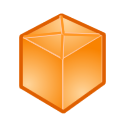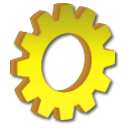﻿ LitDev API

LitDev Extension API

 LD3DView LDArray LDBits LDBlueTooth LDCall LDChart LDClient LDClipboard LDColours LDCommPort LDController LDControls LDCursors LDDataBase LDDateTime LDDebug LDDialogs LDDictionary LDEffect LDEmail LDEncryption LDEvents LDFastArray LDFastShapes LDFigures LDFile LDFocus LDftp LDGraph LDGraphicsWindow LDHID LDIcon LDImage LDInline LDIOWarrior LDList LDLogic LDMath LDMathX LDMatrix LDMusic LDNetwork LDPhysics LDProcess LDQueue LDRegex LDResources LDScrollBars LDSearch LDServer LDSettings LDShapes LDShell LDSort LDSound LDSpeech LDStatistics LDStopwatch LDText LDTextWindow LDTimer LDTranslate LDUnits LDUtilities LDVector LDWaveForm LDWebCam LDWindows LDxml LDZip

LDBitsBitwise logic to store binary flags in a single number as bits.
A 32 bit number is used internally.
This is like a 32 dimension array of 1s and 0s stored in single number.
The bits (1 to 32) are indexed from 1.

 AndBitsGetBitGetBitsNotOrBitsSetBitUnsetBitXOrBitsAndBits(var1,var2)Logically And 2 numbers.
var1 The first number.
var2 The second number.
returns The And number (where both input bits are set).

GetBit(var,bit)Get the bit value in a number.
var The number to test.
bit A bit to test (1 to 32).
returns 0 (unset) or 1 (set).

GetBits(var)Get an array of bit values.
var The number to get the bits.
returns A 32 dimension array of bits (0 or 1).

Not(var)Logically Not a number.
var The number to Not.
returns The Not number (all bits reversed).

OrBits(var1,var2)Logically Or 2 numbers.
var1 The first number.
var2 The second number.
returns The Or number (where either input bits are set).

SetBit(var,bit)Set a bit in a number.
var The number to set the bit.
bit A bit to set (1 to 32).
returns The modified number with bit set.

UnsetBit(var,bit)Unset a bit in a number.
var The number to unset the bit.
bit A bit to unset (1 to 32).
returns The modified number with bit unset.

XOrBits(var1,var2)Logically XOr 2 numbers.
var1 The first number.
var2 The second number.
returns The XOr number (where exclusively either input bits are set).